Lakhmir Singh & Manjit Kaur: Carbon And Its Compounds, Solutions- 2

# Lakhmir Singh & Manjit Kaur: Carbon And Its Compounds, Solutions- 2 - Science Class 10

(Page No : 223)

Question 39:
(a) What are hydrocarbons ? Explain with examples.
(b) Explain the meaning of saturated and unsaturated hydrocarbons with two examples each.
(c) Give the names and structural formulae of one saturated cyclic hydrocarbon and one unsaturated cyclic hydrocarbon.
(d) Give one example of a hydrocarbon, other than pentane, having more than three isomers.
(e) How many isomers of the following hydrocarbons are possible ?
(i) C3H8 (ii) C4H10 (iii) C5H12 (iv) C6H14
Solution :

(a) A compound made up of hydrogen and carbon only is called a hydrocarbon (Hydrogen + Carbon= Hydrocarbon).

For exampe: methane (CH4), ethane (C2H6), ethene (C2H4), and ethyne (C2H2), all are hydrocarbons as they are made up of only two elements: carbon and hydrogen.
(b) Saturated Hydrocarbons: These are the ones in which the carbon atoms are connected by only single bonds. They are also known as alkanes.
Example: Methane (CH4) and ethane (C2H6)
Unsaturated Hydrocarbons: These are the ones in which two carbon atoms are connected by a double bond or a triple bond.
Example: Ethene (C2H4) and ethyne (C2H2)
(c) Saturated cyclic hydrocarbon: Cyclohexane, C6H12
Unsaturated cyclic hydrocarbon: Benzene, C6H6
(d) Hexane, C6H14
(e)
(i) None (ii) Two (iii) Three (iv) Five

(Page No : 224)

Question 60:
A solid element X has four electrons in the outermost shell of its atom. An allotrope Y of this element is used as a dry lubricant in machinery and also in making pencil leads.
(a) What is element X ?
(b) Name the allotrope Y.
(c) State whether allotrope Y is a good conductor or non-conductor of electricity.
(d) Name one use of allotrope Y (other than lubrication and pencil leads)
(e) Name two other allotropes of element X.
Solution :

(a) Element X : Carbon
(b) Allotrope Y: Graphite
(c) Y is a good conductor of electricity.
(d) Y is used for making graphite electrodes or carbon electrodes in dry cells.
(e) Allotropes of X: Diamond and buckminsterfullerene

Question 61:
Two organic compounds A and B have the same molecular formula C6H12. Write the names and structural formulae :
(a) if A is a cyclic compound
(b) if B is an open chain compound
(c) Which compound contains single bonds as well as a double bond ?
(d) Which compound contains only single bonds ?
Solution :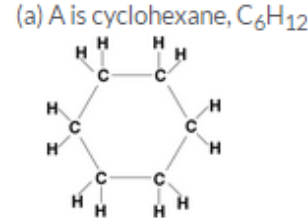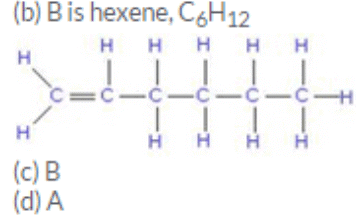Question 62:
The solid element A exhibits the property of catenation. It is also present in the form of a gas B in the air which is utilised by plants in photosynthesis. An allotrope C of this element is used in glass cutters.
(a) What is element A ?
(b) What is the gas B ?
(c) Name the allotrope C.
(d) State another use of allotrope C (other than in glass cutters).
(e) Name another allotrope of element A which exists as spherical molecules.
(f) Name a yet another allotrope of element A which conducts electricity.
Solution :

(a) Element A: Carbon
(b) Gas B: Carbon dioxide
(c) Allotrope C: Diamond
(d) Used for making jewellery
(e) Buckminsterfullerene
(f) Graphite

Question 63:
An element E exists in three allotropic forms A, B and C. In allotrope A, the atoms of element E are joined to form spherical molecules. In allotrope B, each atom of element E is surrounded by three other E atoms to form a sheet like structure. In allotrope C, each atom of element E is surrounded by four other E atoms to form a rigid structure.
(a) Name the element E.
(b) What is allotrope A ?
(c) What is allotrope B ?
(d) What is allotrope C ?
(e) Which allotrope is used in making jewellery ?
(f) Which allotrope is used in making anode of a dry cell ?
Solution :

(a) Element E: Carbon
(b) Allotrope A: Buckminsterfullerene
(c) Allotrope B: Graphite
(d) Allotrope C: Diamond
(e) C
(f) B

Question 64:
You are given the following molecular formulae of some hydrocarbons :
C5H8; C7H4; C6H6; C5H10; C7 H12; C6H12
(a) Which formula represents cyclohexane as well as hexene ?
(b) Which formula represents benzene ?
(c) Which three formulae represent open chain unsaturated hydrocarbons having double bonds ?
(e) Which two formulae represent unsaturated hydrocarbons having triple bonds ?
(f) Which three formulae can represent cyclic hydrocarbons ?
Solution :

(a) C6H12
(b) C6H6
(c) C7H14;
C5H10; C6H12
(d) C5H8;
C7H12
(e) C7H14;
C5H10; C6H12

(Page No : 225)

Question 65:
Which of the following compounds can have a triple bond ?
C2H4, C3H4, C3H6
Solution :

C3H4

Question 66:
Write the molecular and structural formula of a cyclic hydrocarbon whose molecule contains 8 atoms of carbon.
Solution :
Molecular formula: C8H16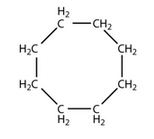Question 67:
What is the molecular formula and structural formula of a cyclic hydrocarbon whose one molecule contains 8 hydrogen atoms ?
Solution :

Molecular formula: C4H8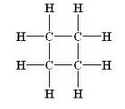Question 68:
Write the molecular formula of : (i) an alkane (ii) an alkene, and (iii) an alkyne, each having 20 carbon atoms.
Solution :

(i) C20H42
(ii) C20H40 (iii) C20H38

Question 69:
Which of the following compounds can have a double bond ?
C4H10; C5H8; C5H10
Solution :

C5H10

Question 70:
Which of the following hydrocarbons is unsaturated ?
C3H4; C2H6
Solution :

C3H4

(Page No : 239)

Question 1:
Write the molecular formula of ethanol.
Solution :

C2H5OH

Question 2:
What is the next higher homologue of methanol (CH3OH) ?
Solution :

Ethanol (C2H5OH)

Question 3:
Identify the functional group present in the following compound and name it according to IUPAC system :
CH3OH

Solution :
Alcohol group;
Methanol

Question 4:
Give the common name and IUPAC name of the simplest aldehyde.
Solution :

Common name:
formaldehyde
IUPAC name: methanal

Question 5:
What is the common name of methanal ?
Solution :

Formaldehyde

Question 6:
Write the names of the following functional groups :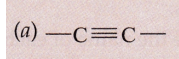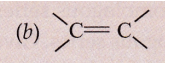Solution :
(i) Alkyne
(ii) Alkene

Question 7:
Name the simplest ketone.
Solution :

Propanone

Question 8:
What is the common name of propanone ?
Solution :

Acetone

Question 9:
Write the IUPAC names of the following :
(i) CH3COCH3 (ii) CH3COCH2CH3
Solution :

(i) Propanone
(ii) Butanone

(Page No : 240)

Question 10:
Write the name and chemical formula of the simplest organic acid.
Solution :

Formic acid; HCOOH

Question 11:
Write the IUPAC names, common names and formulae of the first two members of the homologous series of carboxylic acids.
Solution :

 IUPAC name Common name Formula (i) Methanoic acid Formic Acid HCOOH (ii) Ethanoic acid Acetic acid CH3COOH

Question 12:
What is the common name of : (a) methanoic acid, and (b) ethanoic acid ?
Solution :

(a) Methanoicacid – Formic acid
(b) Ethanoic acid – Acetic acid

Question 13:
Draw the structures for the following compounds :
(a) Ethanoic acid (b) Propanoic acid
Solution :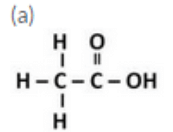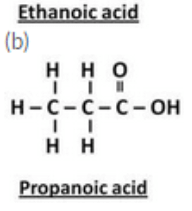Question 14:
Give the common names and IUPAC names of the following compounds :
(a) HCOOH (b) CH3COOH

Solution :

 Formula IUPAC name Common name (i) HCOOH Methanoic acid Formic acid (ii) CH3COOH Ethanoic acid Acetic acid

Question 15:
Give the name and structural formula of one homologue of HCOOH.
Solution :

Ethanoic acid; CH3COOH

Question 16:
Write the formulae of: (a) methanoic acid, and (b) ethanoic acid.
Solution :

(a) Methanoic acid: HCOOH
(b) Ethanoic acid: CH3COOH

Question 17:
Give the common name and IUPAC name of C2H5
Solution :

Common name: Ethyl alcohol
IUPAC name: Ethanol

Question 18:
Give the IUPAC name of the following compound :
C3H7OPI

Solution :
Propanol, C3H7OH

Question 19:
Give the name and structural formula of one member of the following :
Alcohols
Solution :

Ethanol; C2H5OH

Question 20:
Give IUPAC names of the following compounds :
(a) C4H9OH (b) C5HnOH
Solution :

(a) Butanol, C4H9OH
(b) Pentanol, C5H11OH

Question 21:
What is the common name of methanol ?
Solution :

Methyl alcohol

Question 22:
What is the difference between two consecutive homologues :
(i) in terms of molecular mass ?
(ii) in terms of number and kind of atoms per molecule ?
Solution :

(i) 14 u
(ii) Two consecutive homologues differ by 1 carbon atom and 2 hydrogen atoms in their molecular formulae.

Question 23:
What type of fuels :
(a) burn with a flame ?
(b) burn without a flame ?
Solution :

(a) Fuels which vaporise on heating, burn with a flame.
(b) Fuels which do not vaporise on heating, burn without a flame.

Question 24:
State whether the following statement is true or false :
The minimum number of carbon atoms in a ketone molecule is two.
Solution :

False

Question 25:
Fill in the following blanks with suitable words :
(a) The next higher homologue of ethanol is……….
(b) The next homologue of C2H5OH is……….
(c) The next higher homologue of ethane is………..
(d) The functional group present in ethanol is……….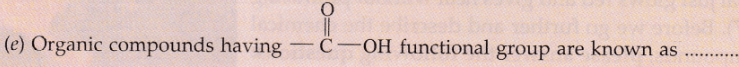Solution :
(a) Propanol
(b) C3H7OH
(c) Propane
(d) -OH (alcohol)
(e) Carboxylic acids

Question 26:
(a) Give the general name of the class of compounds having the general formula CnH2n-2.Write name of the first member of this homologous series.
(b) The general formula of a homologous series of carbon compounds is CnH2n. Write the molecular formulae of the second and fourth members of the series.
(c) Write the molecular formulae of the third and fifth members of homologous series of carbon compounds represented by the general formula CnH2n+2.
Solution :

(a) Alkynes, CnH2n-2
First member: Ethyne
(b) Second member: C3H6
Fourth member: C5H10
(c) Third member: C3H8
Fifth member: C5H12

Question 27:
(a) Give the names and structural formulae of the next two higher homologues of methane.
(b) The molecular formula of a hydrocarbon is C10H18. Name its homologous series.
(c) Select the hydrocarbons which are members of the same homologous series. Give the name of each series.
C5H10 ; C3H8; C6H10; C4H10; C7H12; C8H16
Solution :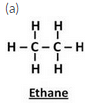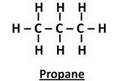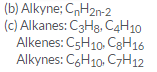Question 28:
(a) Give the molecular formula of one homologue of each of the following :
(i) C3H6 (ii) C2H6 (iii) C2H2
(b) What is the difference in the molecular mass of any two adjacent homologues ?
(c) By how many carbon atoms and hydrogen atoms do any two adjacent homologues differ ?
Solution :

(a) (i) C4H8 (ii) C3H8
(iii) C3H4
(b) 14 u
(c) 1 carbon atom and 2 hydrogen atoms i.e. a CH2 group.

The document Lakhmir Singh & Manjit Kaur: Carbon And Its Compounds, Solutions- 2 | Science Class 10 is a part of the Class 10 Course Science Class 10.
All you need of Class 10 at this link: Class 10

## Science Class 10

78 videos|509 docs|153 tests

## FAQs on Lakhmir Singh & Manjit Kaur: Carbon And Its Compounds, Solutions- 2 - Science Class 10

 1. What are carbon compounds?Ans. Carbon compounds are organic compounds that contain carbon atoms bonded to other elements, such as hydrogen, oxygen, nitrogen, sulfur, etc. These compounds are the basis of life and are found in various forms, including carbohydrates, proteins, fats, and nucleic acids.
 2. What is the importance of carbon compounds?Ans. Carbon compounds are essential for life as they form the building blocks of all living organisms. They are involved in various biological processes, including metabolism, energy production, and structural support. Carbon compounds also play a crucial role in industries, such as the production of fuels, plastics, pharmaceuticals, and dyes.
 3. How are carbon compounds classified?Ans. Carbon compounds can be classified into different categories based on their molecular structure and functional groups. Some common classifications include alkanes, alkenes, alkynes, alcohols, aldehydes, ketones, carboxylic acids, esters, amines, and amides. These classifications help in understanding the properties and reactions of carbon compounds.
 4. What are some examples of carbon compounds?Ans. There are numerous examples of carbon compounds. Some common examples include methane (CH4), ethane (C2H6), ethanol (C2H5OH), acetic acid (CH3COOH), glucose (C6H12O6), proteins, carbohydrates, fats, and DNA. These compounds have diverse functions and are present in our daily lives.
 5. How do carbon compounds contribute to environmental pollution?Ans. Carbon compounds, especially those derived from fossil fuels, contribute to environmental pollution. When these compounds are burned or released into the atmosphere, they can lead to the formation of greenhouse gases, such as carbon dioxide (CO2) and methane (CH4), which contribute to climate change. Additionally, carbon compounds can also cause air pollution and water pollution if not properly treated or disposed of.

## Science Class 10

78 videos|509 docs|153 testsExplore Courses for Class 10 examSignup to see your scores go up within 7 days! Learn & Practice with 1000+ FREE Notes, Videos & Tests.
10M+ students study on EduRev
Track your progress, build streaks, highlight & save important lessons and more!
Related Searches

,

,

,

,

,

,

,

,

,

,

,

,

,

,

,

,

,

,

,

,

,

,

,

,

;